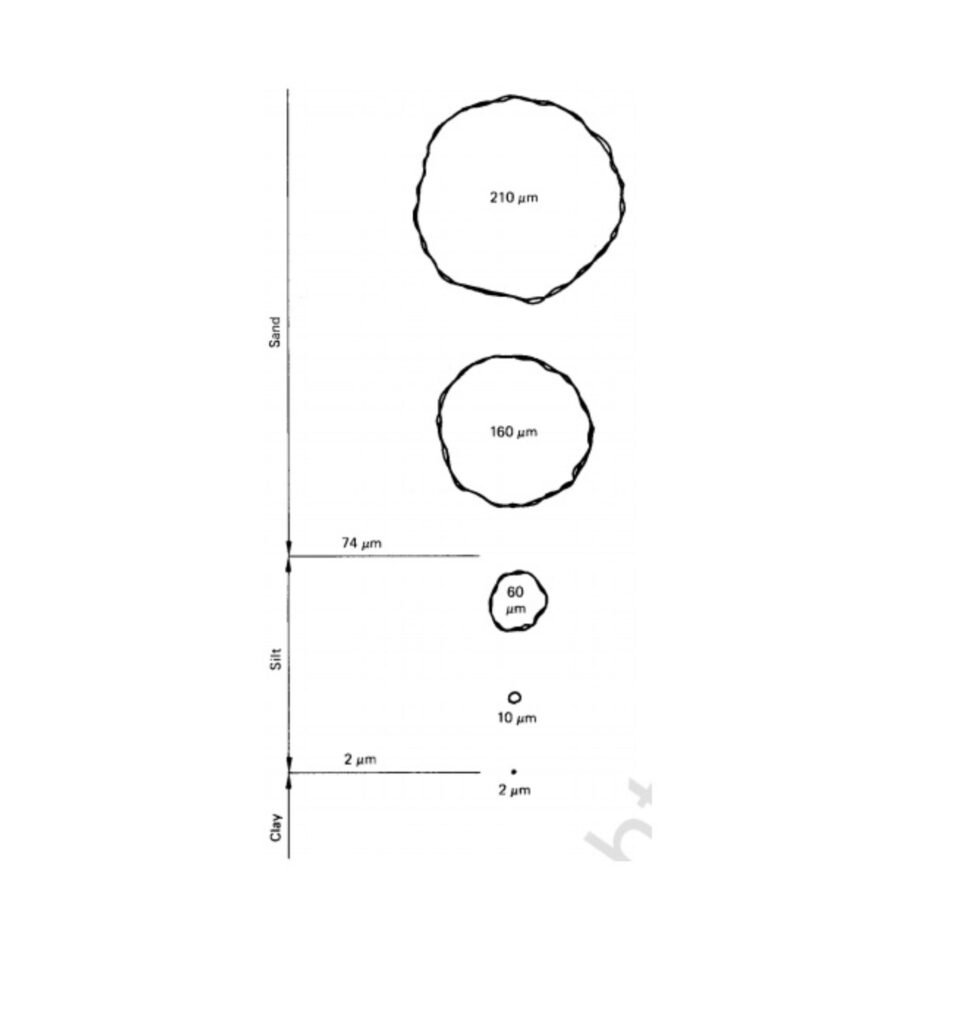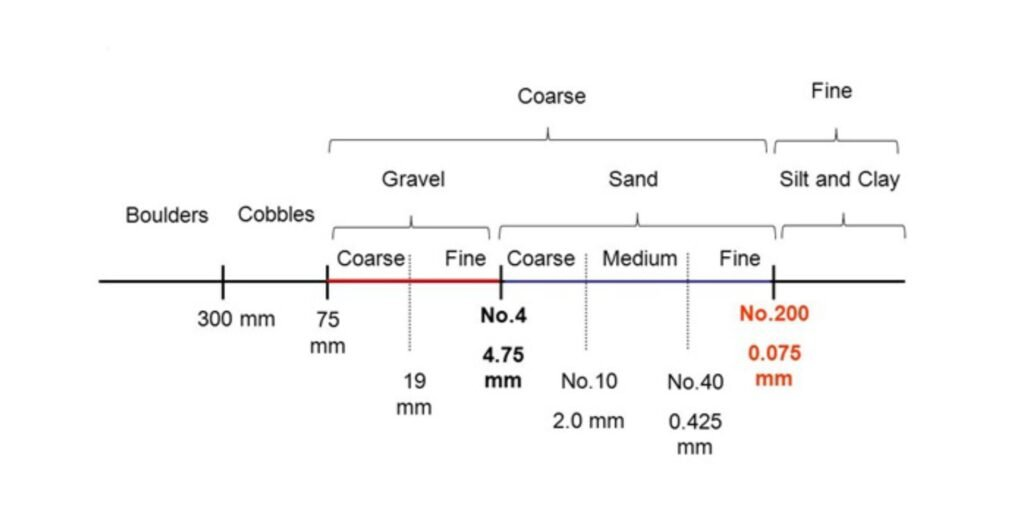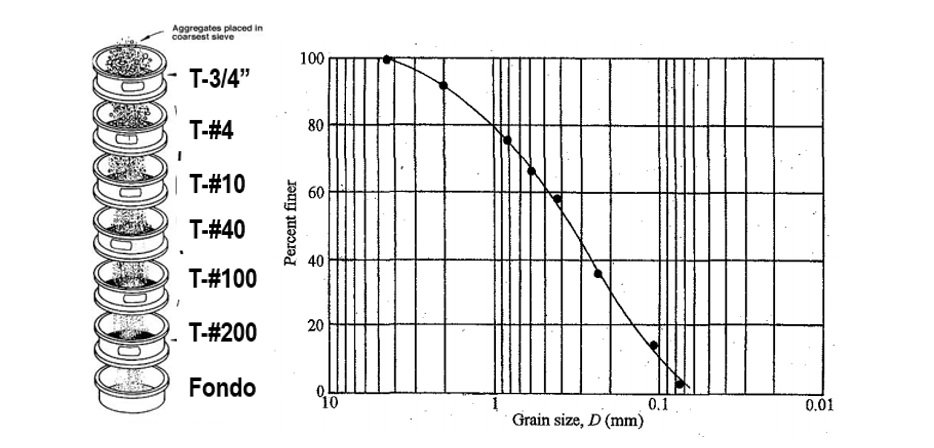## "If you can't explain it simply, you don't understand it well enough" (Albert Einstein)## Sieve grain size distribution analysis

The sieve test is one of the most important tests in Geotechnical Engineering, as it is used to get a fairly accurate idea of the particle size distribution of a soil sample. In this post we will review some important points of the sieve particle size test. Do you want to know more? Continue reading...

### Soil size distribution test

Soil size distribution analysis consists of separating and classifying by size the particles that make up a soil sample. This classification is carried out by determining the quantity of grains of different sizes, expressed as a percentage of the total weight of the sample.

A particularity of soils is that, in general, the sizes of the particles that compose them vary in a wide range, from large particles (larger than 4.75 mm and up to 75 mm) such as gravels, to particles that cannot be seen with the naked eye, such as silts (which have sizes smaller than 0.074 mm) and clays (sizes smaller than 0.002 mm).

In addition, a very important fact in practice is that such a large size distribution can be observed in a single soil sample. To illustrate this, in Figure 1 we see a scale representation of different soil particles, which is very useful to observe at a glance the differences between the particle sizes.Figure 1 Soil particle sizes. Scale figure (Source: Mitchell & Soga, 2005).

On the other hand, Figure 2 shows the denomination given to soil particles according to their size, considering the criteria established in the Unified Soil Classification System, which is the most widely used system around the world for geotechnical studies.Figure 2 Grain particle sises according to USCS.

For practical purposes, soil particle size is defined as the aperture of a sieve with the closest square mesh through which the particle passes. If the particle is less than 0.074 mm, the size will then be the diameter of a sphere of specific gravity equal to that of the soil, descending in distilled water with the same velocity as the actual soil particle.

From the above, it follows that the particle size test can be performed by:

1. Sieve análisis, for coarse particles.
2. Hydrometer analysis, for fine-grained soils (sizes les than 0,074 mm).
• Combined

Another important aspect to consider is that in order to identify and classify fine particles smaller than 0.074 mm, it is necessary to perform Atterberg limit tests (which we have already discussed in this blog), so as to distinguish between silts and clays.

### What does sieve particle size analysis consist of?

Sieve test is performed to analyze the particle size distribution of coarse soils, with sizes greater than 0.074 mm. Basically, the test consists of shaking a soil sample through a set of sieves, and determining the mass of material retained on each sieve. Thus, by comparing this mass retained on each sieve with the total size of the sample, the percentage retained on each sieve can be determined and, by difference with respect to 100%, the passing percentage.

The result of the analysis is presented graphically in the so-called particle size distribution curve. In general, a good spacing of the particle diameters in this curve will be obtained if a set of sieves with openings of approximately half of the previous coarsest sieve is used. Thus, in practice it is common to use the sieves shown in Figure 3.Figure 3 Typical sieves and particle size distribution curve for sieve tests.

Of course, a very important aspect to take into account is that the sample to be tested must be representative, and usually obtained through a quarting process (depending on the size of the sample).

Having an idea of how the sieve test is carried out and how the results are presented, I present some general comments, which may help to better understand some aspects of the test.

1. What does the sieve number "n" mean? This is a very common concern regarding sieve identification. The "n" number refers to the number of squares the sieve mesh has in a linear inch. Thus, sieve #10, for instance, has 10 squares in 1 inch, while sieve #200 has 200 squares.
2. The particle size test is typically performed on an oven-dried sample, in order to prevent the finer particles from adhering to the coarser ones, affecting their size
3. In some soils that form clods, a rubber mallet must be used to break them up. Why a rubber mallet? Simply because if an iron hammer is used, some soil particles may break, thus disturbing the sample.
4. In the case of predominantly fine soils, the lime removal process can become very complicated. That is why, in these cases, the sample should be washed through the #200 sieve (which would eliminate the fine part, of sizes smaller than 0.074 mm), and then the sample should be dried in the oven and the sieve test will be performed on this material.

This is a fairly simple test, but very useful in Geotechnical Engineering. In future posts we will go a little deeper into the hydrometric test, to analyze the size distribution of fine particles, and about some parameters (such as uniformity and curvature coefficients) that help to better interpret the size distribution of a soil sample.

### References

• Das, B. (2002) “Soil Mechanics Laboratory Manual”. Fifth Edition. Engineering Press, Inc. California, USA.
• Head, K. (1980) “Manual of Soil Laboratory Testing – Volume 1: Soil Classification and Compaction Tests”. Pentech Press. London, UK.
• Mitchell, J.K. & Soga, K. (2005) “Fundamentals of Soil Behavior”. Third Edition. John Wiley & Sons, Inc. New Jersey, USA.